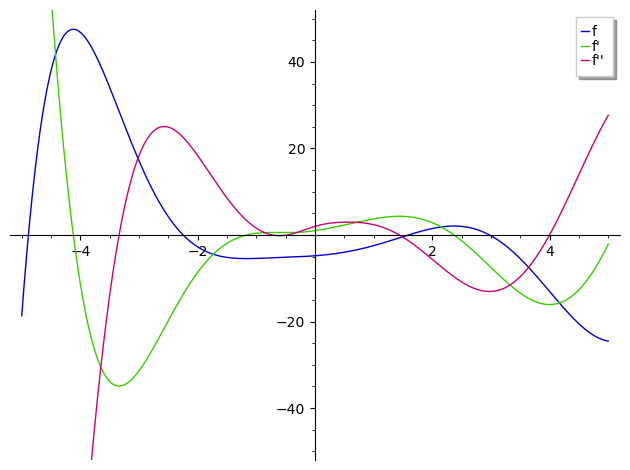# problem with solving equation and finding the maximum and minimumAnonymous

Hi, i'm trying to find the Root of a function, but i could only get one point while there should be another 3 points, i also tried to find the Inflection, maximum and minimum points, but error appeared, though when i change the equation iw works well. Please someone help me it's driving me crazy since yesterday!

Thank you very much

reset()
P.<x> = QQ[]
f=log(1+x^2)-((exp(-x))*(cos(x)))+((x^2)*(sin(x)))-(log(sqrt(1991)))
find_root(f, -5,5)
f1 = f.derivative()
f2 = f1.derivative()
show(f1)
show(f2)
minimax_candidates = set([e for (e, mult) in f1.roots()])
minimax_candidates
Inflection = set([e for (e, mult) in f2.roots()])
Inflection
minimax = list(minimax_candidates.difference(Inflection))
minimax = [(e, f(e)) for e in minimax]
minimax

edit retag close merge delete

Sort by » oldest newest most voted

First and foremost, your expression of interest involves transcendental function not defined in polynomial rings. Working in suh a ring is pointless.

Second : there is no known general way to solve mixed-expressions (in your case, yourexpression uses polynomials, trig and exp functions) in closed form. Trying to find numerical approximations of your roots is useful, but will limit your conclusions to the interval you're exploring.

Third, from find_root? (emphases mine) :

Numerically find a root of "f" on the closed interval [a,b] (or [b,a]) if possible, where "f" is a function in the one variable. Note: this function only works in fixed (machine) precision, it is not possible to get arbitrary precision approximations with it.

What you neeed is a function recursively exploring your search interval for numerical roots and returning the (possibly empty) list of such zeros. One possible implementation :

def find_roots(ex, a, b):
"""
(Recursively) numerically explore the interval [a, b] fpr zeroes of
the single variable expression f, and return the (posibly empty) list
of such zeroes.
This will never return if this list is infinite
(e. g. sin(1/x) between 0 and 1).
"""
if len(ex.variables())!=1 : raise RuntimeError(
"""
find_roots :
the expression to solve must have exactly one variable
""")
if (a>b): a, b=b, a
epsilon=1e-4
try:
r1 = find_root(ex, a, b)
return find_roots(ex, a, r1-epsilon) +\
[r1] +\
find_roots(ex, r1+epsilon, b)
except RuntimeError:
return []


NOTE : The timings reported below are abnormal, and I can't reproduce them : running the same code in a newly-started Sage is much faster. Discard the timings and timing-related temarks...

Let's put this to work : zeroes :

sage: %time Zeroes = find_roots(f, -5, 5) ; Zeroes
CPU times: user 34.4 s, sys: 24 ms, total: 34.4 s
Wall time: 34.4 s
[-4.8863727269823904,
-2.248189884926029,
1.591391293749397,
2.9760183676051764]


Extrema :

sage: %time Extrema = find_roots(f.diff(x), -5, 5)
CPU times: user 26.4 s, sys: 8.01 ms, total: 26.4 s
Wall time: 26.4 s
sage: %time Maxima = [u for u in Extrema if f.diff(x,2)(x=u)<0] ; Maxima
CPU times: user 8.55 s, sys: 12 ms, total: 8.56 s
Wall time: 8.57 s
[-4.120692958580293, 2.369318406384495]
sage: %time Minima = [u for u in Extrema if f.diff(x,2)(x=u)>0] ; Minima
CPU times: user 8.43 s, sys: 6 µs, total: 8.43 s
Wall time: 8.43 s
[-1.167409895786979]


Check that we have no pathological cases (simultaneous nullity of f' and f'') :

sage: set(Extrema)-(set(Maxima)|set(Minima))
set()


Inflection points :

sage: %time Inflections = find_roots(f.diff(x, 2), -5, 5) ; Inflections
CPU times: user 1min 41s, sys: 23 µs, total: 1min 41s
Wall time: 1min 41s
[-3.340415675479655,
-0.7259000686402566,
-0.4752900420219365,
1.4375916288836839,
3.997537922882414]


These results appear consistent with the plots on the interval :One notes that this search isn't especially fast. You may try to define f, f' and f'' as Cython functions : with appropriate type declaration and unique computation of the numerical constants, this should give you a notable speed boost.

Similarly, it might be interesting to reimplement find_roots as a generator (with some interesting edge cases...).

All of this is left to you as an exercise (and an exercise in laziness for me :-)).

more